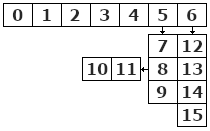# SetTableSchema

SetTableSchema changes the schema of a table.
```unsigned __int64 SetTableSchema (
unsigned int tableID,
const CODBPP::Schema *schema = NULL,
const unsigned int *fieldMapping = NULL
);```

### Parameters

 tableID the table ID defining the table's handle. schema pointer to a valid schema. fieldMapping An array of int values allowing this method to map field from there old schema to the new - eg. fieldMapping = 2, will move the value in the first field of the old table into the third field of the new table. Sub fields are appended to the end so that and so no.### Return Values

If the method succeeds, the return value is zero else see error codes for more details.

### Remarks

• If fieldMapping parameter is missing, than SetTableSchema will map the first fixed field to the first fixed field, the second fixed field to the second and so on, plus the first variable field to the first variable field and so on.
• SetTableSchema is not part of the transaction.
• When schema equals NULL SetTableSchema attempts a rebuild and/or upgrade for the table file to its current standard and this will also open the table.
Example Use
```#define TABLE_ONE 1
int length;
CODBPP database;
CODBPP::Schema *schema;
if((error = database.OpenDatabase(u"YourDatabase")) == NO_ERROR
&& (error = database.BeginTransaction(CODBPP::EXCLUSIVE)) == NO_ERROR
&& (error = database.OpenTable(TABLE_ONE)) == NO_ERROR
&& (error = database.GetTableSchema(TABLE_ONE,NULL,&length)) == NO_ERROR){
schema = (CODBPP::Schema*) new BYTE[length];
if((error = database.GetTableSchema(TABLE_ONE,schema)) == NO_ERROR){
//removes the last index if there is one
if(schema.indexCount > 0){
schema.indexCount--;
error = database.SetTableSchema(TABLE_ONE,schema);
}
}
delete [] (LPBYTE)schema;
}
if(error && database.GetErrorMessage(&message) == NO_ERROR)
MessageBox(message);
database.EndTransaction();```

### Also See

GetTableSchema